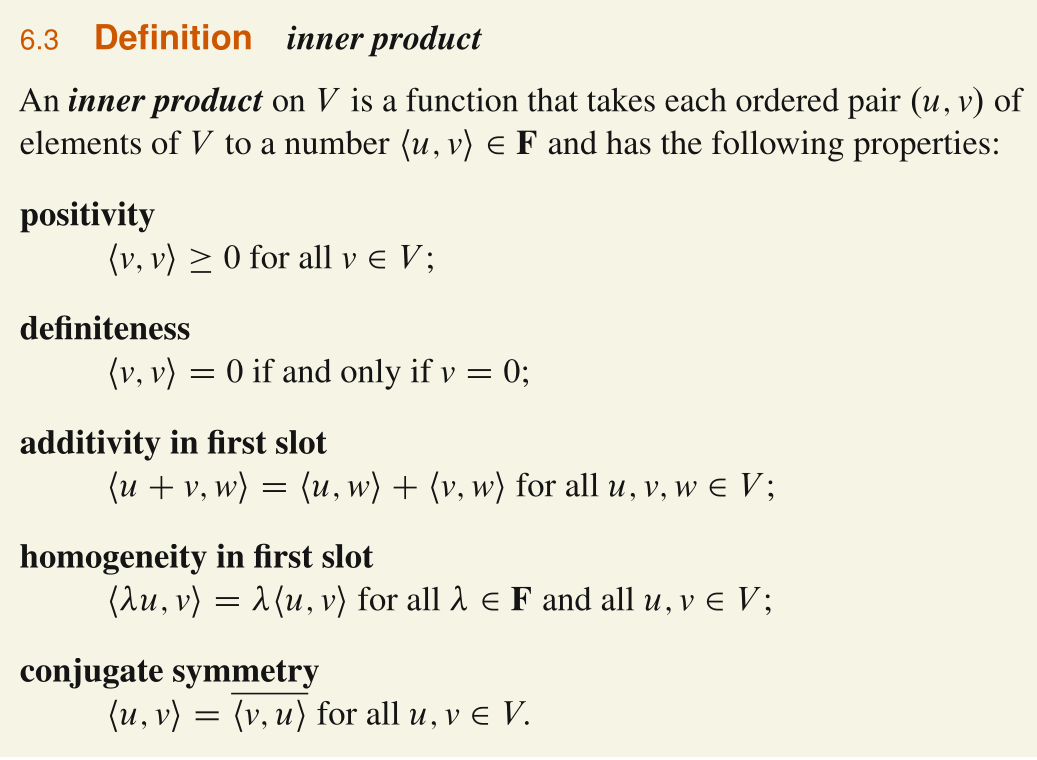# [GSLA ch-1,2,3] Vectors, Vector Spaces and Matrix

### 1.1 Vectors and Linear Combination

column vector $v = \begin{bmatrix}v_1\\ v_2\end{bmatrix}$

vector addition $v = \begin{bmatrix}v_1\\ v_2\end{bmatrix}$ and $w = \begin{bmatrix}w_1 \\ w_2\end{bmatrix}$ add to $v + w = \begin{bmatrix} v_1+w_1 \\v_2+w_2 \end{bmatrix}$

scalar multiplication $2v = \begin{bmatrix} 2v_1 \\ 2v_2 \end{bmatrix}$

linear combination $cv+dw$

### 3.1 Spaces of Vectors

Vector Space

Definition: A vector space is a set $V$ on which two operations + and · are defined, called vector addition and scalar multiplication.

following the conditions for all $u$, $v$, $w \in V$ and $c$, $d \in R$

• The operation + (vector addition) must satisfy the following conditions (V is an abelian group):

• Closure: The sum $u + v$ belongs to $V$.
1. Commutative law: $u + v = v + u$
2. Associative law: $u + (v + w) = (u + v) + w$
3. Additive identity: The set $V$ contains an additive identity element, denoted by 0, where $0 + v = v$ and $v + 0 = v$.
4. Additive inverses: The equations $v + x = 0$ and $x + v = 0$ have a solution $x$ in $V$, called an additive inverse of $v$, and denoted by $-v$.
• The operation · (scalar multiplication) is defined between vectors and real numbers (or any other Field with addition + and multiplication x), must satisfy the following conditions:

• Closure: The product $c · v$ belongs to $V$.
1. Distributive law: $c · (u + v) = c · u + c · v$
2. Distributive law: $(c+d) · v = c · v + d · v$
3. Associative law: $c · (d · v) = (cd) · v$
4. Unitary law: $1 · v = v$

Examples

• The groups $R^n$ and $C^n$ are vector spaces over $R$, with scalar multiplication given by
$$λ(x_1 , . . . , x_n ) = (λx_1 , . . . , λx_n ),$$

• The ring $R[X]_n$ of polynomials of degree at most n with real coefficients is a vector space over $R$, with scalar multiplication $λ·P (X)$ is given by
$$λ · P (X) = λa_m X_m + λa_{m−1} X_{m−1} + · · · + λa_1 X + λa_0 .$$

Counterexamples

• $(x_1, x_2, …) + (y_1, y_2, … ) = (x_1 + y_2, x_2 + y_1,… )$
• $c \cdot (x_1, x_2, …) = (0, 0, …..)$

subspace

Let $V$ be a vector space, with operations + and ·, and let $W$ be a subset of $V$. Then $W$ is a subspace of $V$ if and only if the following conditions hold

• Nonempty: zero vector always belongs to $W$
• Closure: If $u$ and $v$ are any vectors in $W$, then $c · u + d · v \in W$

the “vectors” in vector space could be anything### 3.4 Independence, Basis and Dimension

Linear Independence

$x_1v_1 +x_2v_2 + … + x_nv_n = 0$ only happens when all x’s are zero

Span

A set of vectors spans a space if their linear combinations fill the space.

Basis

The basis vectors are linearly independent and they span the space.

Dimenision

The dimension of a space is the number of vectors in every basis.

### 2.1 Vectors and Linear Equations

Solving two EquationsRow picture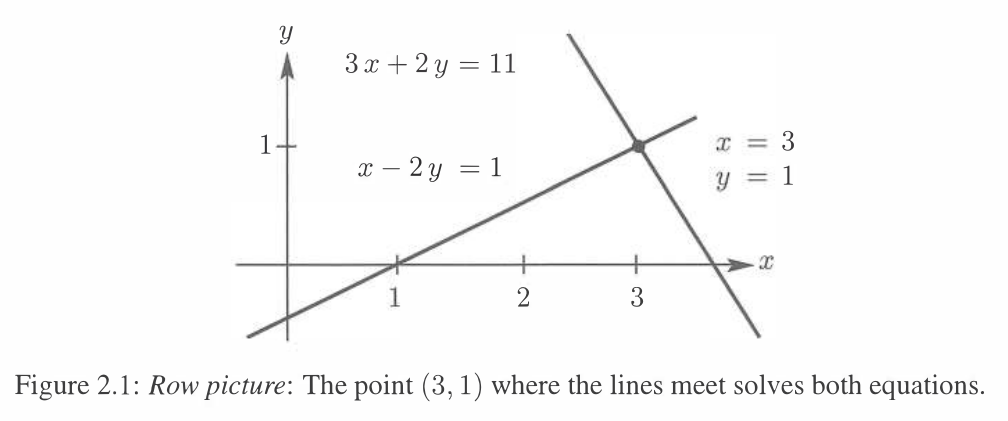Column picture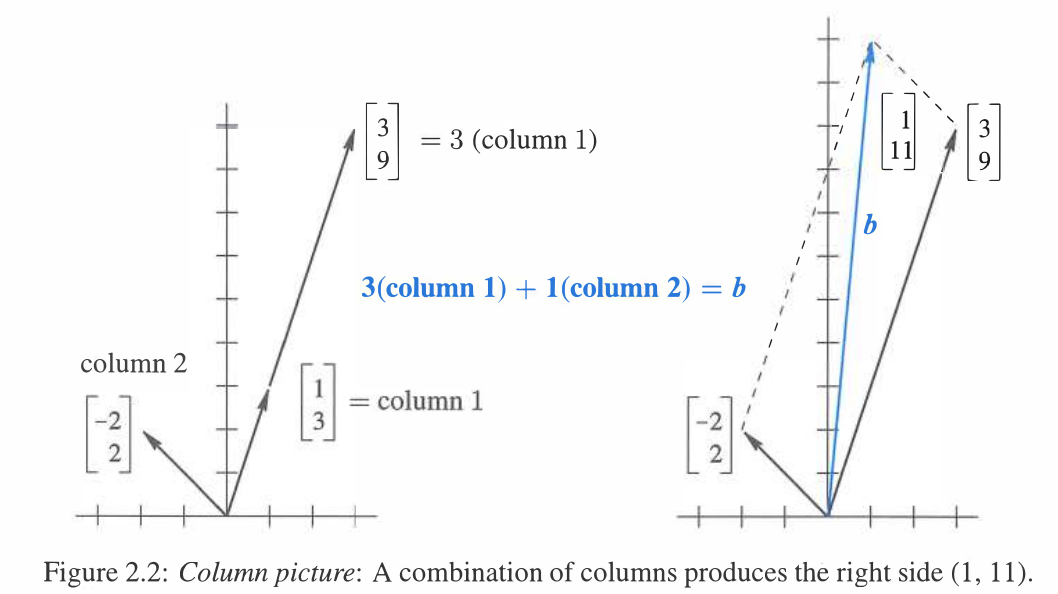Represent as coefficient matrix multiplying scalar vector### 2.2 The Idea of Elimination

Multiply the first equation by $a_{21}/ a_{11}$ and subtract from the second: then $x_1$ is eliminated

The corner entry $a_{11}$ is the first “pivot” and the ratio $a_{21}/a_{11}$ is the first “multiplier”.

### 2.3 Elimination Using Matrices

to solve $Ax=b$, we apply $EAx = Eb$

According to elimination steps, $E$ is lower triangular with 1 in all diagonals and negative values under diagonal

### 2.4 Rules for Matrix Operations

Fundamental Law of Matrix Multiplication: $(AB)C = A(BC)$

left multiplication $BA$ represents row combination of $A$
right multiplication $AB$ represents column combination of $A$

### 2.5 Inverse Matrices

$A^{-1}A = AA^{-1} = I$

$(AB)^{-1} = B^{-1}A^{-1}$

solving augmented matrix $A^{-1} [ \ A \ \ I\ ] = [\ I\ \ A^{-1}\ ]$

### 3.1 & 3.2 Column Space and Null Space of A

column space

• linear combination of column vectors $(R^m)$
$$Ax = b \\ x_1\begin{bmatrix} \\ a_1 \\ \\ \end{bmatrix} + x_2\begin{bmatrix} \\ a_2 \\ \\ \end{bmatrix}… = \begin{bmatrix} \\ b \\ \\ \end{bmatrix}$$

null space

• linear combination of solution vector $(R^n)$
$$Ax = 0 \\ c\begin{bmatrix} \\ x_1 \\ \\ \end{bmatrix} + d\begin{bmatrix} \\ x_2 \\ \\ \end{bmatrix}… = 0$$

Rank

GSLA

• number of pivots = number of independent columns (rows) = dimension of column (row) space

LADR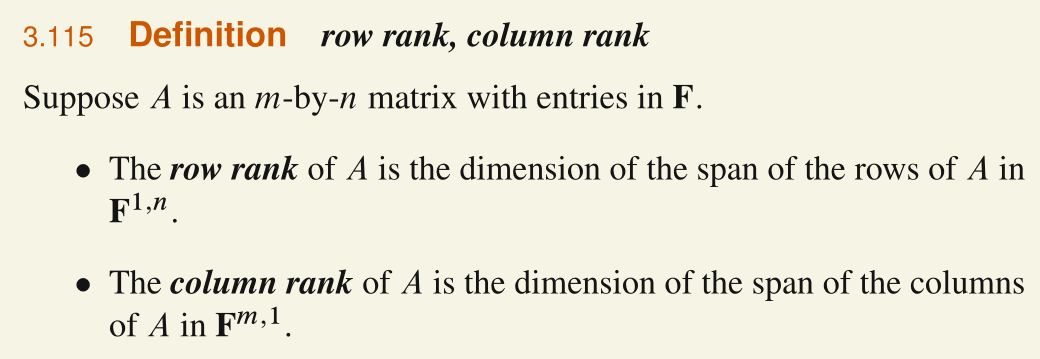Math-Deep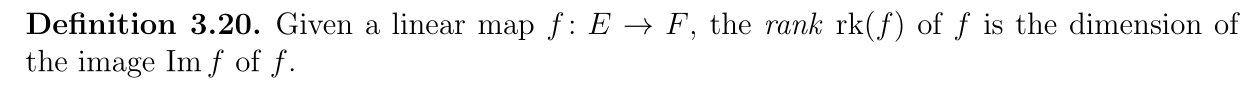### 3.3 Elimination: The Big Picture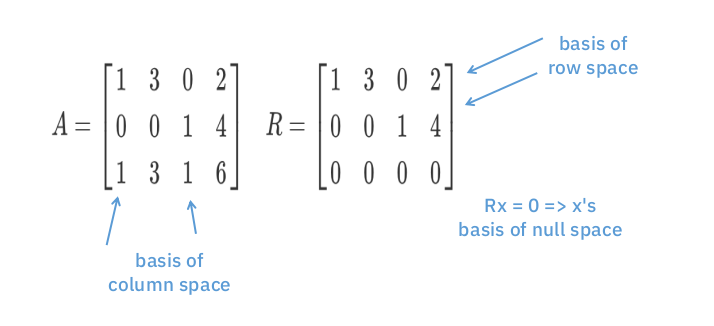### 3.3 The Complete Solution to Ax = b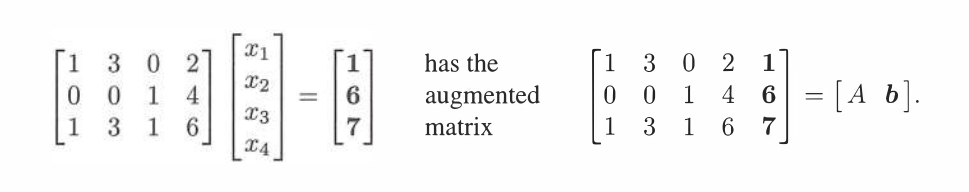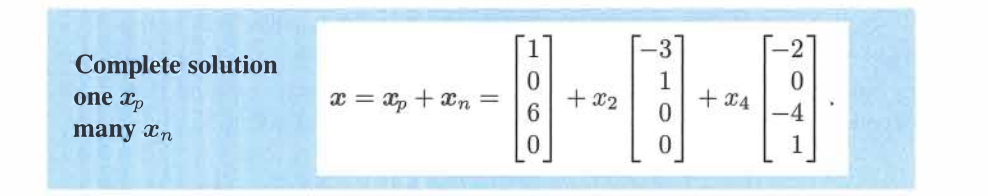### 3.5 Dimensions of the Four Subspaces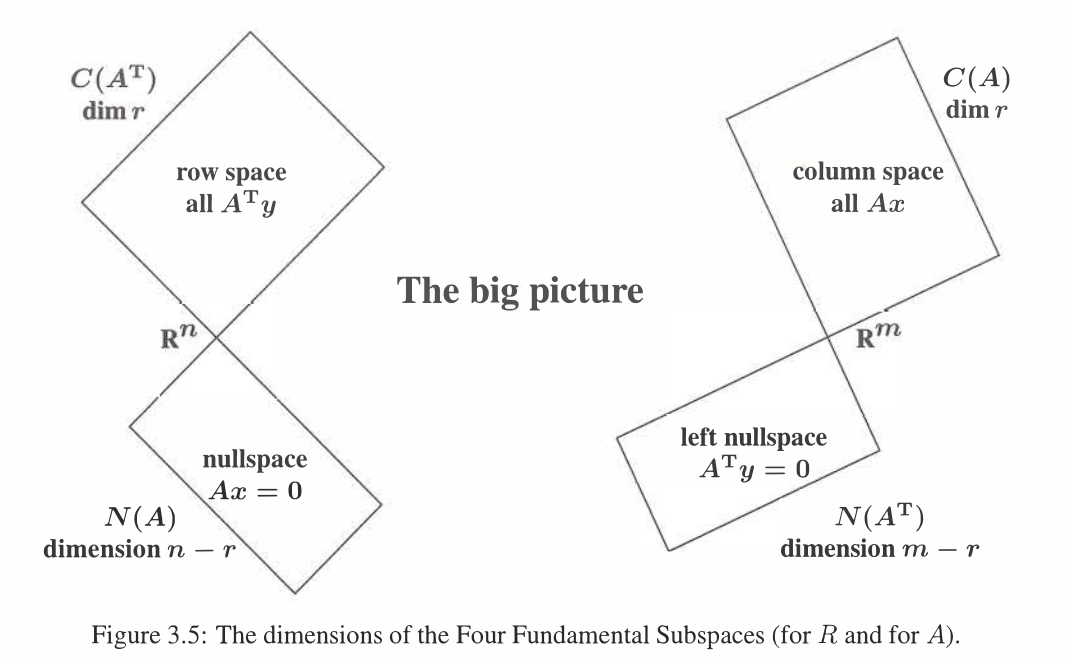### 1.2 Lengths and Dot Products